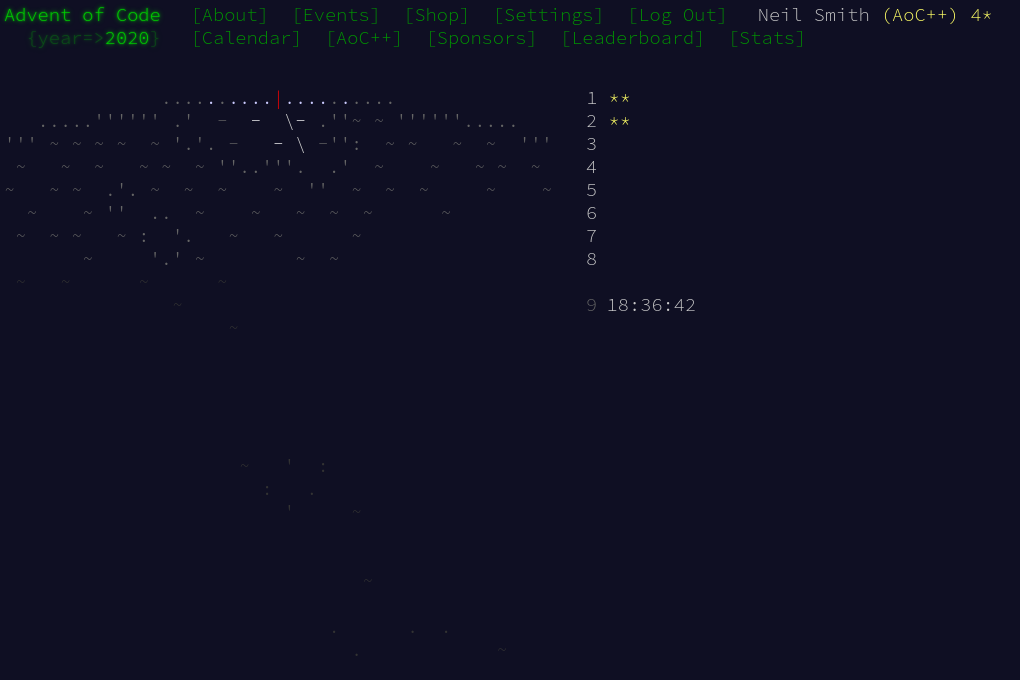December 9, 2020

# Advent of Code 2020 days 1 and 2

First two days: warming up.A bit late to start the event this year, as life got in the way a bit. But better late than never.

## Day 1

A simple warm-up task. Read a text file, convert to numbers, and find a few numbers that match a criterion. I did entertain the idea of using a Set of numbers, but decided a List as good enough. I thought aboutdoing something clever with using the first number (or numbers) to calculate the last one, then checking of that calculated number existed. But there are only a few numbers, so a brute force search of the Cartesian product of the numbers is good enough.

List comprehensions to the rescue! This is the entirety of the code (but note the use of the visible type application when converting the strings to numbers). I order the numbers in the comprehension so I only find each solution once. I also assume that all the given numbers are distinct.

main :: IO ()
main =
let nums = map (read @Int) $lines numStrs print$ head $part1 nums print$ head $part2 nums part1 nums = [ x * y | x <- nums , y <- nums , x < y , x + y == 2020 ] part2 nums = [ x * y * z | x <- nums , y <- nums , z <- nums , x < y , y < z , x + y + z == 2020 ]  ## Day 2 A bit more data structuring here. I decided to represent the policies as a list of records, defined like this: data Policy = Policy { lower :: Int , upper :: Int , character :: Char , password :: String } deriving (Show, Eq, Ord)  I used Megaparsec again to parse the input file. It's a bit more cumbersome than using a regular expression, but much of it is boilerplate. I find the final parser is much more readable, mainly because all the parts have names, unlike the various groups in a regex. -- Parse the input file type Parser = Parsec Void Text sc :: Parser () sc = L.space space1 CA.empty CA.empty lexeme = L.lexeme sc integerP = lexeme L.decimal symb = L.symbol sc hyphenP = symb "-" colonP = symb ":" characterP = alphaNumChar <* sc passwordP = some alphaNumChar <* sc policiesP = many policyP policyP = Policy <$> integerP <* hyphenP <*> integerP <*> characterP <* colonP <*> passwordP


The enforcement of rules is relatively direct as well. The're both taking the length of the set of policies after applying a filter. For part 1, count the number of occurrences of the given character, then check it's in range. For part 2, check the character at the two positions and check that exactly one of them matches. (For Booleans, not-equal-to, /=, is the same as XOR.)

part1 = length . filter inRange
where nCharsPresent p = length \$ filter (== (character p)) (password p)
inRange p = (nCharsPresent p >= (lower p)) && (nCharsPresent p <= (upper p))

part2 = length . filter matches

matches p = ((pw!!l) == c) /= ((pw!!u) == c)